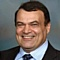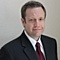# Project Management Central

Please login or join to subscribe to this thread
Decision Tree Analysis questionAnonymous
Can someone help make sure I am doing this correctly?

Given the following Decision Tree, perform decision tree analysis to determine which is best to do, make or buy a software application as part of a development project? Remember that this requires the use of EMV.

Decision Tree Outline
• System
o Make
? 90% - Profit [\$50,000]
? 30% - Simple [\$23,000]
? 70% - Difficult [\$34,000]
o Buy
? 35% - Minor Changes [\$17,000]
? 65% - Major Changes [\$35,000]
? 90% - Profit [\$35,000]

Should you make or buy the system?
For make product:
? Profit: 0.9 x \$50,000 = \$45,000
? Simple: 0.3 x \$23,000 = \$6,900
? Difficult: 0.7 x \$34,000 = \$23,800
? Total EMV =

For buy product:
? Minor changes: 0.35 x 17,000 = \$5,950
? Major changes = 0.65 x 35,000 = \$22,750
? Profit = 0.9 x 35,000 = \$31,500
? Total EMV =

Note: When calculating the EMV, make sure profits are calculated as positive (+) values and losses are calculated as negative (-) values.
Sort By:Network:1136It's much easier to take a decision based on scope. You can't really predict the cost of customisations. That calculation is pure academic. There is no way to calculate the percentages that accurate.
If the scope is not covered at least 90% then is better to go for a customises solution because the cost of adapting a COTS will spiral exponentially.Network:1506A few questions:

1. Can you clarify what is meant by simple vs. difficult?
2. If there is a 90% likelihood of profit in both situations, what happens with the remaining 10% - no profit?
3. Do you mean profit or revenue as the other items are costs?
4. Is the cost of buying the system built into the major & minor changes?

KironNetwork:18I'm not sure where profit comes into this problem. If this is for internal use, then cost is the only consideration (performance being equal). If this is for sale to external customers, I'm going to make a big assumption: what the customer gets doesn't matter if made vs. bought.

If the above is true, this looks like a decision tree with 7 nodes. The root node is a decision of make vs. buy. There are two chance nodes that are children of the root node, one for make and one for buy. Finally, there are four end nodes, two for each chance nodes.

The EMV of the make chance node is: (.3*-23000) + (.7*-34000) = -\$30700

The EMV of the buy chance node is: (.35*-17000) + (.65*-35000) = -\$28700

Since we are dealing with costs, we would choose the least negative EMV which is buy. Therefore, the EMV of the root node is -\$28700.

Please login or join to reply

Content ID:
ADVERTISEMENTS
 We're going to have the best-educated American people in the world. - Dan Quayle
ADVERTISEMENT